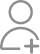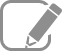Take Tuition from the Best Tutors

•Affordable fees
•1-1 or Group class
•Flexible Timings
•Verified Tutors

Search in

# 5(X^2+Y^2+Z^2)=4(XY+YZ+ZX) then find X:Y:ZFollow 29Answer3 XYZ

IITIAN DHARMADAS (M.Sc. from IIT GUWAHATI)

Solving the equation you will get (2x-y)^2+(2y-z)^2+(2z-x)^2=0 Since sum of three square number is zero so they are individually equal to 0. So 2x-y=0; 2y-z=0; 2z-x=0 From these three equation we get x=y=z=0

1:1:1

10

Experienced (10+ Y) & Certified Mathematics Assistant Professor from Uttaranchal University, Dehradun

X=Y=Z

Computer Professional Teacher with more than 15 years of experience in Computer Science

X:Y:Z=1:1:1

0:0:0

A Teacher with 20 years of experience.

In this condition, there is no exact value given for the equation. Hence we cannot solve it without a given state. We cannot find a specific answer for each variable as there are no values given. I also tried the trial and error method, but the answer keeps fluctuating. We might have to create assumptions,...

In this condition, there is no exact value given for the equation. Hence we cannot solve it without a given state. We cannot find a specific answer for each variable as there are no values given. I also tried the trial and error method, but the answer keeps fluctuating. We might have to create assumptions, and then we can solve it.

Tutor with an experience in teaching Mathematics since 6 years.

5(x2 + y2 + z2) = 4(xy + yz + zx) or, 5x2 + 5y2 + 5z2 = 4xy + 4yz + 4zx or, 4x2 + 4y2 + 4z2 + x2 + y2 + z2 = 4xy + 4yz + 4zx or, (4x2 – 4xy + y2) + (4y2 – 4yz + z2) + (4z2 – 4zx + x2) = 0 or, (2x - y)2 + (2y - z)2 + (2z - x)2 = 0 We know that, since sum of squares is 0, each square...

5(x2 + y2 + z2) = 4(xy + yz + zx)

or, 5x2 + 5y2 + 5z2 = 4xy + 4yz + 4zx

or, 4x2 + 4y2 + 4z2 + x2 + y2 + z2 = 4xy + 4yz + 4zx

or, (4x2 – 4xy + y2) + (4y2 – 4yz + z2) + (4z2 – 4zx + x2) = 0

or, (2x - y)2 + (2y - z)2 + (2z - x)2 = 0

We know that, since sum of squares is 0, each square must be equal to 0.

Therefore,
(2x - y)2 = (2y - z)2 = (2z - x)2 = 0

or, (2x - y) = (2y - z) = (2z - x) = 0

Therefore,

2x = y, 2y = z, 2z = x.

Thus converting all terms in respect to ‘x’ we get,
y = 2x
z = x/2
Therefore,
x : y : z = x : 2x : x/2
= 1 : 2 : ½
= 2 : 4 : 1

Answer:      x : y : z = 2 : 4 : 1

Related Questions5 days ago
20Now ask question in any of the 1000+ Categories, and get Answers from Tutors and Trainers on UrbanPro.com

Related Lessons

Find Tuition near you

Looking for Tuition ?

Learn from the Best Tutors on UrbanPro

Are you a Tutor or Training Institute?

Join UrbanPro Today to find students near you
X

### Looking for Tuition Classes?

The best tutors for Tuition Classes are on UrbanPro

• Select the best Tutor
• Book & Attend a Free Demo
• Pay and start Learning### Take Tuition with the Best Tutors

The best Tutors for Tuition Classes are on UrbanProUrbanPro.com is India's largest network of most trusted tutors and institutes. Over 55 lakh students rely on UrbanPro.com, to fulfill their learning requirements across 1,000+ categories. Using UrbanPro.com, parents, and students can compare multiple Tutors and Institutes and choose the one that best suits their requirements. More than 7.5 lakh verified Tutors and Institutes are helping millions of students every day and growing their tutoring business on UrbanPro.com. Whether you are looking for a tutor to learn mathematics, a German language trainer to brush up your German language skills or an institute to upgrade your IT skills, we have got the best selection of Tutors and Training Institutes for you. Read more